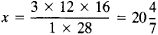# Chain Rule

Also found in: Dictionary, Wikipedia.
Related to Chain Rule: implicit differentiation

## chain rule

[′chān ‚rül]
(mathematics)
A rule for differentiating a composition of functions: (d/dx) ƒ (g (x)) = ƒ′(g (x))· g ′(x).
McGraw-Hill Dictionary of Scientific & Technical Terms, 6E, Copyright © 2003 by The McGraw-Hill Companies, Inc.
The following article is from The Great Soviet Encyclopedia (1979). It might be outdated or ideologically biased.

## Chain Rule

a method given in old arithmetic textbooks for converting the measures of one system into the measures of another system by means of a third system.

Suppose, for example, we want to know the number of vershki equal to 3 feet if 1 foot is equal to 12 inches and 28 inches are equal to 16 vershki. To apply the chain rule, we write the elements of the problem in the following form:

 x vershki 3 feet 1 foot 12 inches 28 inches 16 vershki

The required number of vershki is obtained by dividing the product of the numbers in the right-hand column by the product of the known numbers in the left-hand column:References in periodicals archive ?
If f [member of] [C.sup.1](R, R) and g : T [right arrow] R is delta differentiable, then the time scales chain rule, see [5, Theorem 1.90], states that
By using the chain rule, the update law of [W.sub.rj] is
In , the authors used the following chain rule [MATHEMATICAL EXPRESSION NOT REPRODUCIBLE IN ASCII] to convert a fractional differential equation with Jumarie's modification of Riemann-Liouville derivative into its classical differential partner.
Osler, Leibniz rule, the chain rule and taylors theorem for fractional derivatives [Ph.D.
Using Lemmas 7 and 8 and the chain rule we find that the derivative of A is
Concept of a function, derivative, integral, chain rule, arclength.
When [partial derivative]E([theta]) / [partial derivative][p.sub.k] = [partial derivative]E[theta] / [partial derivative]y [partial derivative]y / [partial derivative][p.sub.k] is determined with chain rule, this gives partial derivative]y / [partial derivative][p.sub.k] = [[theta].sub.k][x.sup.Pk] ln(x).
In the function notation, the chain rule indicates: h'(x) = f(g(x)) x g(x).
The readers are invited to find analogous pictures for the chain rule, related rates, and integration by parts.
This third edition offers three new chapters on fixed- or finite-memory filters, use of the chain rule from calculus for filter initialization, and use of a bank of linear sine-wave Kalman filters for estimating the actual frequency of noisy sinusoidal measurements.
Practice the golden supply chain rule: "Treat your suppliers the way you want your customers to treat you."
For available local partial derivatives of the arithmetic operators and intrinsic functions provided by the programming language, the Jacobian of the function at the given arguments can be accumulated by using the chain rule. This technique is known as automatic differentiation of numerical programs.

Site: Follow: Share:
Open / Close